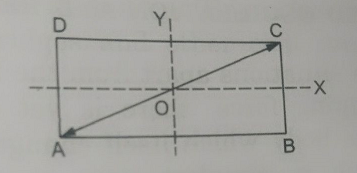# If two mutually perpendicular simple harmonic motion of same amplitude, frequency and having zero phase difference superimpose on a particle then its resultant path will be

4 years ago

If    and

This is a equation of straight line.

Resultant equation of two perpendicular SHM when δ = 0 -

Resultant equation

- wherein

It is a straight line with slope